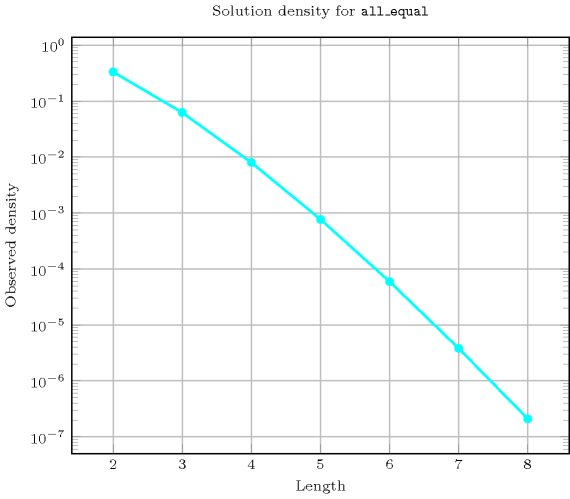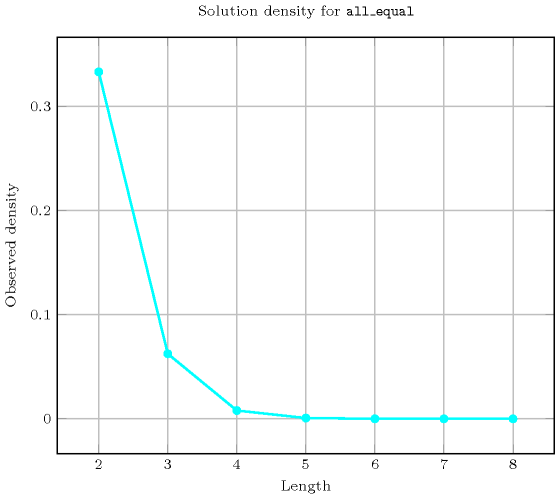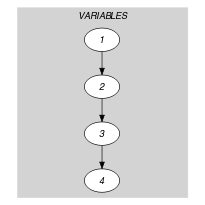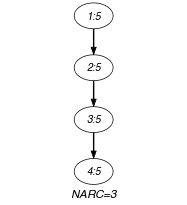## 5.5. all_equal

Origin
Constraint

$\mathrm{𝚊𝚕𝚕}_\mathrm{𝚎𝚚𝚞𝚊𝚕}\left(\mathrm{𝚅𝙰𝚁𝙸𝙰𝙱𝙻𝙴𝚂}\right)$

Synonym

$\mathrm{𝚛𝚎𝚕}$.

Argument
 $\mathrm{𝚅𝙰𝚁𝙸𝙰𝙱𝙻𝙴𝚂}$ $\mathrm{𝚌𝚘𝚕𝚕𝚎𝚌𝚝𝚒𝚘𝚗}\left(\mathrm{𝚟𝚊𝚛}-\mathrm{𝚍𝚟𝚊𝚛}\right)$
Restrictions
 $\mathrm{𝚛𝚎𝚚𝚞𝚒𝚛𝚎𝚍}$$\left(\mathrm{𝚅𝙰𝚁𝙸𝙰𝙱𝙻𝙴𝚂},\mathrm{𝚟𝚊𝚛}\right)$ $|\mathrm{𝚅𝙰𝚁𝙸𝙰𝙱𝙻𝙴𝚂}|>0$
Purpose

Enforce all variables of the collection $\mathrm{𝚅𝙰𝚁𝙸𝙰𝙱𝙻𝙴𝚂}$ to take the same value.

Example
$\left(〈5,5,5,5〉\right)$

The $\mathrm{𝚊𝚕𝚕}_\mathrm{𝚎𝚚𝚞𝚊𝚕}$ constraint holds since all its variables are fixed to value 5.

All solutions

Figure 5.5.1 gives all solutions to the following non ground instance of the $\mathrm{𝚊𝚕𝚕}_\mathrm{𝚎𝚚𝚞𝚊𝚕}$ constraint: ${V}_{1}\in \left[0,6\right]$, ${V}_{2}\in \left[0,2\right]$, ${V}_{3}\in \left[0,2\right]$, ${V}_{4}\in \left[1,4\right]$, $\mathrm{𝚊𝚕𝚕}_\mathrm{𝚎𝚚𝚞𝚊𝚕}$$\left(〈{V}_{1},{V}_{2},{V}_{3},{V}_{4}〉\right)$.

##### Figure 5.5.1. All solutions corresponding to the non ground example of the $\mathrm{𝚊𝚕𝚕}_\mathrm{𝚎𝚚𝚞𝚊𝚕}$ constraint of the All solutions slotTypical
 $|\mathrm{𝚅𝙰𝚁𝙸𝙰𝙱𝙻𝙴𝚂}|>2$ $\mathrm{𝚖𝚒𝚗𝚟𝚊𝚕}$$\left(\mathrm{𝚅𝙰𝚁𝙸𝙰𝙱𝙻𝙴𝚂}.\mathrm{𝚟𝚊𝚛}\right)\ne 0$
Symmetries
• Items of $\mathrm{𝚅𝙰𝚁𝙸𝙰𝙱𝙻𝙴𝚂}$ are permutable.

• All occurrences of a value of $\mathrm{𝚅𝙰𝚁𝙸𝙰𝙱𝙻𝙴𝚂}.\mathrm{𝚟𝚊𝚛}$ can be renamed to any unused value.

Arg. properties

Contractible wrt. $\mathrm{𝚅𝙰𝚁𝙸𝙰𝙱𝙻𝙴𝚂}$.

Counting
 Length ($n$) 2 3 4 5 6 7 8 Solutions 3 4 5 6 7 8 9

Number of solutions for $\mathrm{𝚊𝚕𝚕}_\mathrm{𝚎𝚚𝚞𝚊𝚕}$: domains $0..n$Systems

generalisation: $\mathrm{𝚗𝚟𝚊𝚕𝚞𝚎}$ (a variable counting the number of distinct values is introduced).

specialisation: $\mathrm{𝚎𝚚}$ (equality between just two variables).

Keywords
Cond. implications

$\mathrm{𝚊𝚕𝚕}_\mathrm{𝚎𝚚𝚞𝚊𝚕}\left(\mathrm{𝚅𝙰𝚁𝙸𝙰𝙱𝙻𝙴𝚂}\right)$

with  $|\mathrm{𝚅𝙰𝚁𝙸𝙰𝙱𝙻𝙴𝚂}|>1$

implies $\mathrm{𝚜𝚘𝚖𝚎}_\mathrm{𝚎𝚚𝚞𝚊𝚕}$$\left(\mathrm{𝚅𝙰𝚁𝙸𝙰𝙱𝙻𝙴𝚂}\right)$.

Arc input(s)

$\mathrm{𝚅𝙰𝚁𝙸𝙰𝙱𝙻𝙴𝚂}$

Arc generator
$\mathrm{𝑃𝐴𝑇𝐻}$$↦\mathrm{𝚌𝚘𝚕𝚕𝚎𝚌𝚝𝚒𝚘𝚗}\left(\mathrm{𝚟𝚊𝚛𝚒𝚊𝚋𝚕𝚎𝚜}\mathtt{1},\mathrm{𝚟𝚊𝚛𝚒𝚊𝚋𝚕𝚎𝚜}\mathtt{2}\right)$

Arc arity
Arc constraint(s)
$\mathrm{𝚟𝚊𝚛𝚒𝚊𝚋𝚕𝚎𝚜}\mathtt{1}.\mathrm{𝚟𝚊𝚛}=\mathrm{𝚟𝚊𝚛𝚒𝚊𝚋𝚕𝚎𝚜}\mathtt{2}.\mathrm{𝚟𝚊𝚛}$
Graph property(ies)
$\mathrm{𝐍𝐀𝐑𝐂}$$=|\mathrm{𝚅𝙰𝚁𝙸𝙰𝙱𝙻𝙴𝚂}|-1$

Graph model

We use the arc generator $\mathrm{𝑃𝐴𝑇𝐻}$ in order to link consecutive variables of the collection $\mathrm{𝚅𝙰𝚁𝙸𝙰𝙱𝙻𝙴𝚂}$ by a binary equality constraint.

Parts (A) and (B) of Figure 5.5.2 respectively show the initial and final graph of the Example slot. Since we use the $\mathrm{𝐍𝐀𝐑𝐂}$ graph property, the arcs of the final graph are stressed in bold.

##### Figure 5.5.2. Initial and final graph of the $\mathrm{𝚊𝚕𝚕}_\mathrm{𝚎𝚚𝚞𝚊𝚕}$ constraint(a) (b)